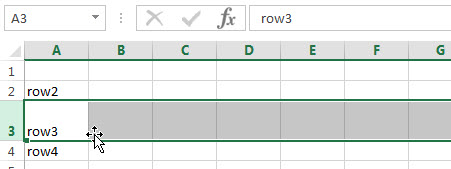## Extract Multiple Match Values into Separate Columns

If you have a few values/items in the excel sheet and you are thinking that with the aid of the “VlOOKUP” function you can look for a specific value, extract it and then put the matching item into the separate column in Ms Excel easily, then congratulations, you are thinking right, but here a problem arises that there isn’t any doubt that by this way you can extract one or two matches into the separate column easily but with the aid of this way you cannot extract multiple matches into separate columns and if you would do that by this way then there are 90% chances that you would 100% get tired of it and can’t complete your task at the right time.

But don’t be worry about it because after carefully reading this article extracting multiple matches into the separate columns would become a piece of cake for you.

So let’s dive into the article to take you out of this fix.

## General Formula:

For extracting multiple matches(items) into seprate columns you can use the  Array Formula which is based upon INDEX and SMALL, which is stated as follows:

`=IFERROR(INDEX(STU_Range,SMALL(IF(CLASS_Range=\$E2, ROW(STU_Range) -MIN (ROW(STU_Range))+1),COLUMNS(\$E\$2:E2))),"")`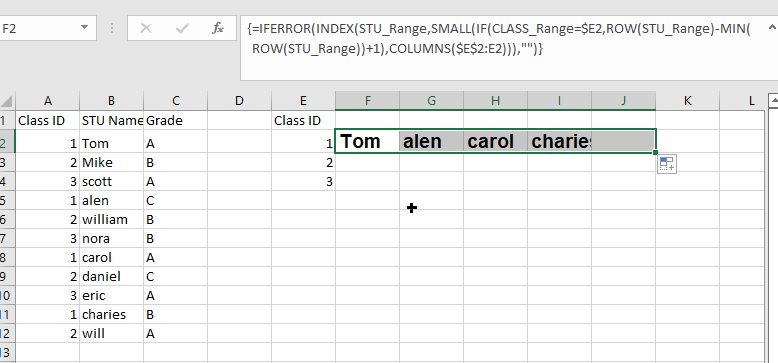## Syntax Explanations:

Before knowing about how to use this formula for getting the work done efficiently, we must understand each syntax which would make it easy for you that how each syntax contributes to extracting multiple matches into the separate columns:

• `IFERROR`: This Function returns a custom result whenever a formula generates an error and returns the expected result when no error is detected.
• `INDEX`: In a range or array, this index function contributes to returning the value at a given position.
• `SMALL`: From the given range of data, this small Function returns the Nth
• `IF`: In Excel, this IF Function contributes to returning two different values, one value for the TRUE result and another for the FALSE result.
• `ROW`: In Excel, this Row function contributes toreturning the row number as a reference.
• `MIN`: From the range of input values, this MIN function contributes to returning the smallest numeric value.
• `Absolute Reference`: The Absolute referenceis nothing but an actual fixed location in a worksheet.
• `COLUMNS`: From a given reference, this Column function contributes to counting the columns.
• `Comma symbol (,)`: This symbol acts as a separator that contributes to separating a list of values.
• `Minus Operator (-)`: This minus symbol contributes to subtracting any two values.
• `Parenthesis ()`: The primary purpose of this parenthesis symbol is to group the various elements.
• `Name`– It represents the input ranges in your worksheet.
• `Plus operator (+)`: This plus symbol adds the values.

## Let’s See How This Formula Works:

To use this array formula for getting the work done, you must enter this formula with Control + Shift + Enter. As soon as you would enter this formula into the first cell, you need to drag it down and across to fill in the other cells.

As you can see in the above screenshot, this formula uses two names ranging: “ CLASS_Range ” and “ STU_Range,” where “ STU_Range ” refers to B2:B12 and on the other hand “ CLASS_Range ” refers to A2:A12.

You would definitely wonder that how this formula works to extract multiple matches into columns? So here is the answer. In this formula, we use the Small Function and INDEX function, which work together.

As the SMALL Function (dynamically constructed by IF) is used to obtain row number corresponding to an “nth match,” so after getting the row number from SMALL Function, this would then pass it into the INDEX function, which returns the value at that row, this is also the main motive of this formula.

The snippet “IF(CLASS_Range=\$E2, ROW(STU_Range) -MIN (ROW(STU_Range))+1” tests the named range “ STU_Range ” for the value in E2. If the value is found, then from an array of relative row numbers, it would return a row number, which is created with:

`=ROW(STU_Range) -MIN (ROW(STU_Range))+1`

The output of this formula is :

`{1;2;3;4;5;6;7;8;9;10;11}`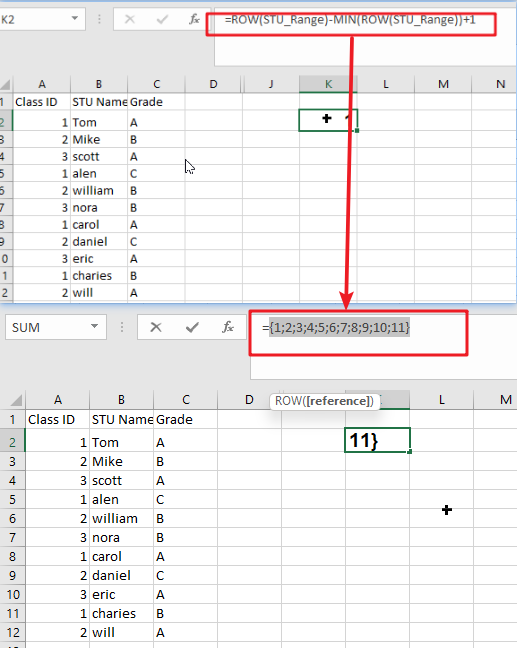Now the final result is an array that would contain the numbers where there is a match, and FALSE where there is not any match found:

`{1;FALSE;FALSE;4;FALSE;FALSE;7;FALSE;FALSE;10;FALSE}`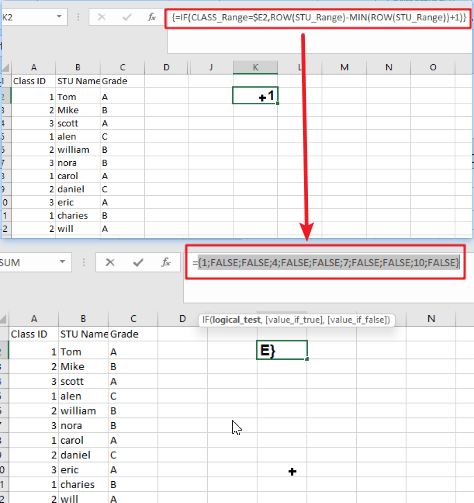Then this array goes into the SMALL Function. By expanding range(Given Below), The k value for SMALL (nth) returns:

`COLUMNS(\$E\$2:E2)`

The SMALL function returns each matching row number, which is then supplied as the row_num to the INDEX function as the array with the range named “ STU_Range.”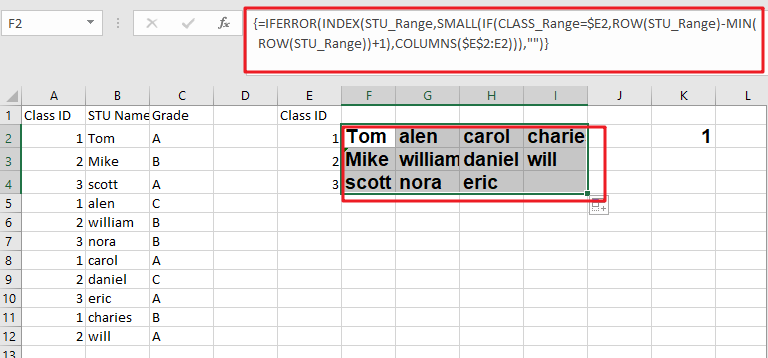Notes:

Now, this question would pop up in your mind that how would it handle the errors? Then whenever the COLUMN would return a value for k that does not exist, the #NUM error would be thrown by the SMALL Function at the next moment. This usually occurs when all the matches have occurred. To tackle the errors, the formula is wrapped up in the Function named “IFERROR,” which would receive the errors and then return an empty string (” “).

### Related Functions

• Excel INDEX function
The Excel INDEX function returns a value from a table based on the index (row number and column number)The INDEX function is a build-in function in Microsoft Excel and it is categorized as a Lookup and Reference Function.The syntax of the INDEX function is as below:= INDEX (array, row_num,[column_num])…
• Excel MATCH  function
The Excel MATCH function search a value in an array and returns the position of that item.The MATCH function is a build-in function in Microsoft Excel and it is categorized as a Lookup and Reference Function.The syntax of the MATCH function is as below:= MATCH  (lookup_value, lookup_array, [match_type])….
• Excel IF function
The Excel IF function perform a logical test to return one value if the condition is TRUE and return another value if the condition is FALSE. The IF function is a build-in function in Microsoft Excel and it is categorized as a Logical Function.The syntax of the IF function is as below:= IF (condition, [true_value], [false_value])….
• Excel ROW function
The Excel ROW function returns the row number of a cell reference.The ROW function is a build-in function in Microsoft Excel and it is categorized as a Lookup and Reference Function.The syntax of the ROW function is as below:= ROW ([reference])….
• Excel SMALL function
The Excel SMALL function returns the smallest numeric value from the numbers that you provided. Or returns the smallest value in the array.The syntax of the SMALL function is as below:=SMALL(array,nth) …
• Excel MIN function
The Excel MIN function returns the smallest numeric value from the numbers that you provided. Or returns the smallest value in the array.The MIN function is a build-in function in Microsoft Excel and it is categorized as a Statistical Function.The syntax of the MIN function is as below:= MIN(num1,[num2,…numn])….
• Excel IFERROR function
The Excel IFERROR function returns an alternate value you specify if a formula results in an error, or returns the result of the formula.The syntax of the IFERROR function is as below:= IFERROR (value, value_if_error)….
• Excel COLUMN function
The Excel COLUMN function returns the first column number of the given cell reference.The syntax of the COLUMN function is as below:=COLUMN ([reference])….

## How to extract word that containing a specific character

This post will guide you how to extract word that containing a specific character using formula in excel. How to get a word that contains a specific character (such as: @, comma, hash, etc.) in a text string in one cell.

## Extract word that containing a specific character

If you want to extract word that contains a hash character in a text string in Cell B1, you can use a combination of the TRIM function, the MID function, the SUBSTITUTE function, the REPT function, the FIND function to create an excel formula as follows:

`=TRIM(MID(SUBSTITUTE(B1," ",REPT(" ",255)),FIND("#",SUBSTITUTE(B1," ",REPT(" ",255)))-100,255))`

Let’s see how this formula works:

= SUBSTITUTE(B1,” “,REPT(” “,255))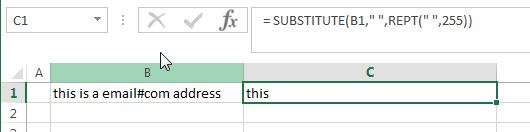The REPT function will return a new text string that contains 255 empty spaces.  And it goes into the SUBSTITUTE function as its new_text argument.  Then the SUBSTITUTE function will replace all empty string with new text value returned by the REPT function.

= FIND(“#”,SUBSTITUTE(B1,” “,REPT(” “,255)))-100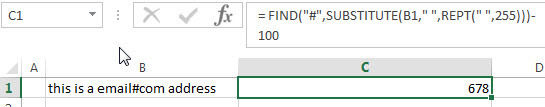This formula will locate the position of the first hash character (#) in a text string that returned by the SUBSTITUTE function.

= MID(SUBSTITUTE(B1,” “,REPT(” “,255)),FIND(“#”,SUBSTITUTE(B1,” “,REPT(” “,255)))-100,255)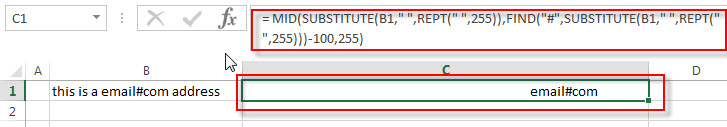This MID function will extract 255 characters from a text string (returned by the SUBSTITUTE function) at a specific position that returned by the FIND function. Then the word that contain hash character will be returned.

=TRIM()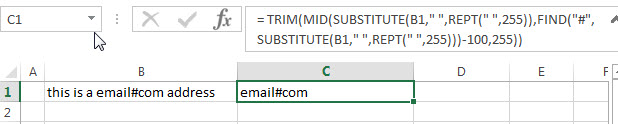The TRIM function removes all spaces from text string returned by the MID function, just leave one space between words.

### Related Formulas

• Split Multiple Lines from a Cell into Rows
If you have multiple lines in a cell and each line is separated by line break character or press “alt + enter” key while you entering the text string into cells, and you need to extract the multiple lines into the separated rows or columns, you can use a combination with the TRIM function, the MID function, the SUBSTITUTE function, the REPT function, the LEN function to create a complex excel formula..…
• Extract word that starting with a specific character
Assuming that you have a text string that contains email address in Cell B1, and if you want to extract word that begins with a specific character “@” sign, you can use a combination with the TRIM function, the LEFT function, the SUBSTITUTE function, the MID function, the FIND function, the LEN function and the REPT function to create an excel formula.…

### Related Functions

• Excel Substitute function
The Excel SUBSTITUTE function replaces a new text string for an old text string in a text string.The syntax of the SUBSTITUTE function is as below:= SUBSTITUTE  (text, old_text, new_text,[instance_num])….
• Excel TRIM function
The Excel TRIM function removes all spaces from text string except for single spaces between words.  You can use the TRIM function to remove extra spaces between words in a string.The syntax of the TRIM function is as below:= TRIM (text)….
• Excel MID function
The Excel MID function returns a substring from a text string at the position that you specify.The syntax of the MID function is as below:= MID (text, start_num, num_chars)….
• Excel FIND function
The Excel FIND function returns the position of the first text string (sub string) within another text string.The syntax of the FIND function is as below:= FIND(find_text, within_text,[start_num])…
• Excel REPT function
The Excel REPT function repeats a text string a specified number of times.The syntax of the REPT function is as below:= REPT  (text, number_times)…

## Excel Columns

How do I insert a new column in excel? How do I delete a column in excel? How to delete multiple columns in Excel workbook? How to hide a column from being displayed in excel? How to unhide the hidden columns in excel? This post will guide you how to Insert/Delete/Hide/Unhide/Sizing columns in Microsoft Excel.

## Insert Column

If you wish to insert a new column in current workbook, just following below steps:

#1 Select one column or a cell that you want to insert the new column and the new column will be inserted on the left side of the selected column or cell. For example: select column B or B1 cell.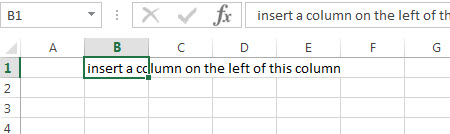#2 Right-click and then click “Insert…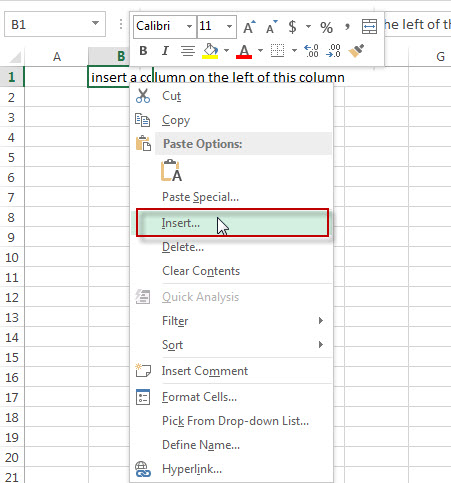#3  A “Insert” window will appear. Then click “Entire column” radio button.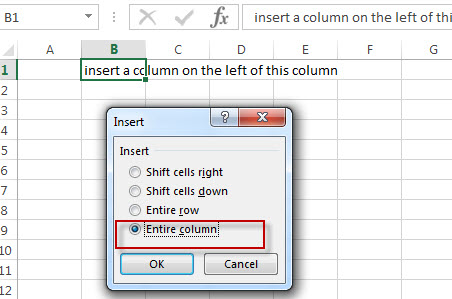#4 Let’ see the result.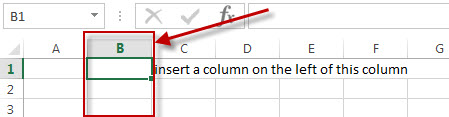## Delete Column

If you wish to delete one column or multiple columns in current workbook, just following below steps:

#1 Select a cell or a column that you want to delete. For example: select column 2 or B2 cell.

#2 Right-click and then click “Delete…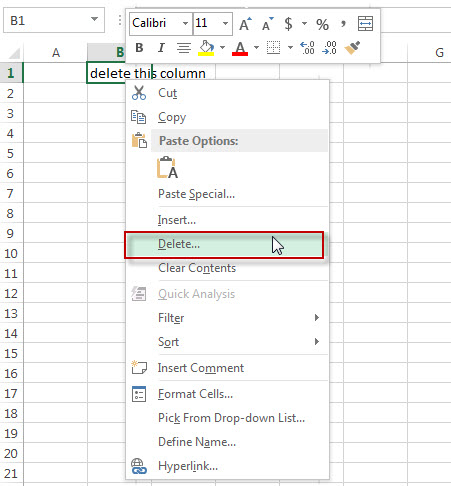#3  A “Delete” window will appear. Then click “Entire row” radio button. Click “OK” button.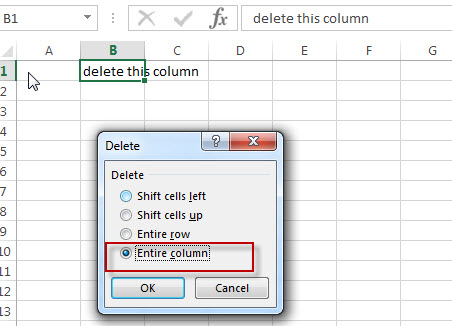#4 Let’ see the result.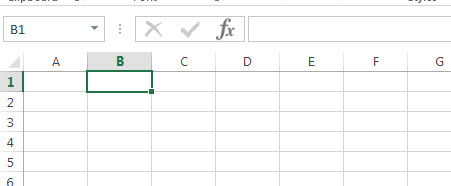Note: if you want to delete multiple columns, you just need to select those columns via clicking column number then right-click on it.  Next, click “Delete”. All columns you selected will be deleted immediately.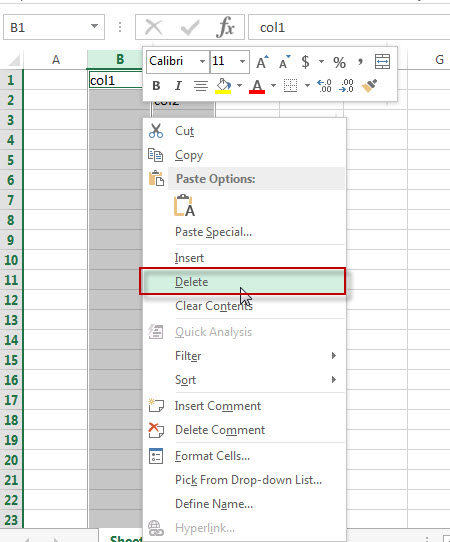## Hide Column

The below steps will explain that how to hide a column in excel.

#1 Select column that you wish to hide via clicking column number.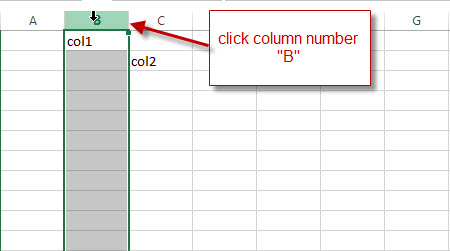#2  Right-click on column B, then click “Hide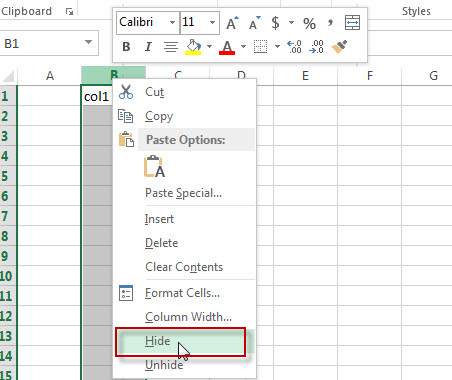#3 Let’s see the result, column B should be hidden.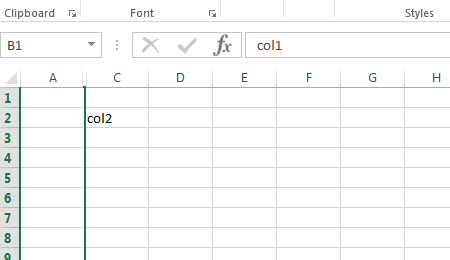## Unhide row

If you wish to unhide the hidden column, please refer to the below steps:

#1  Select the columns that contain the hidden column. For example, column B was hidden, now we need to select Column A and Column C.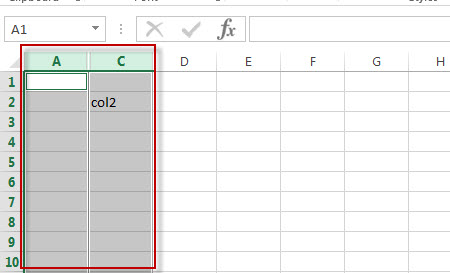#2 Right click and select “Unhide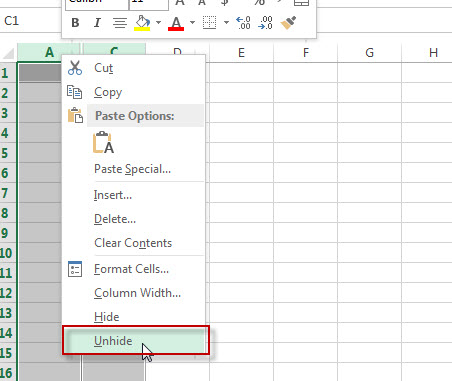#3 The hidden column should be unhidden, let’s see the result.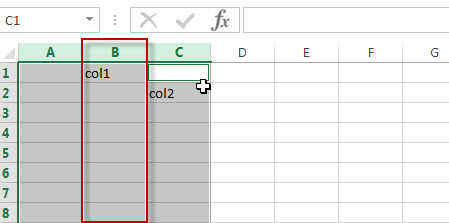## Sizing Column

Sometimes, we wish to change the width of a column in excel, the below steps will guide you how to change the column width.

#1 Select column that you want to change width via clicking column number.

#2 Right-click and then click “Column Width…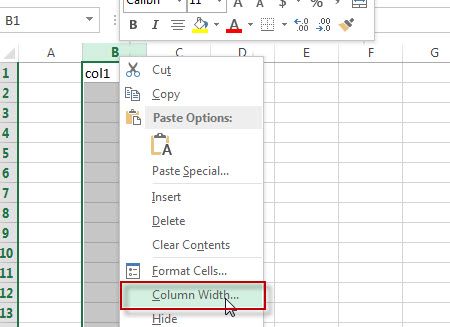#3  A “Column width” window will appear.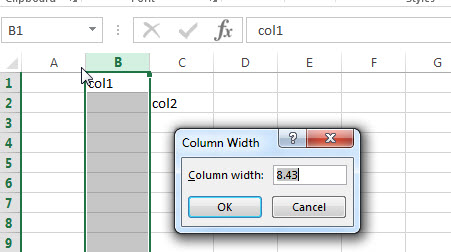#4  Input new value for column width, such as: 20, then click “OK” button.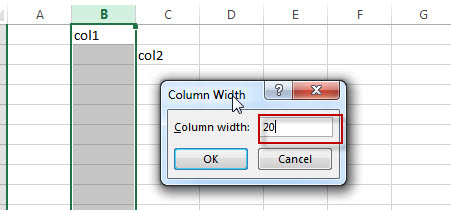#5  Let’s see the result. column 3 should be the new column width.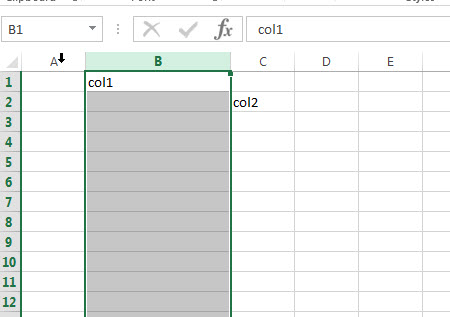## Excel Rows

How do I insert a new row in excel? How do I delete a row in excel? How to delete multiple rows in Excel workbook? How to hide a row from being displayed in excel? How to unhide the hidden rows in excel? This post will guide you how to Insert / Delete/ Hide/ Unhide/ Sizing columns in Microsoft Excel.

## Insert Row

If you wish to add a new row in current workbook, just following below steps:

Step 1# Select one row or a cell that you want to insert the new row and the new row will be inserted above the selected row. For example: Select row 2 or A2 cell.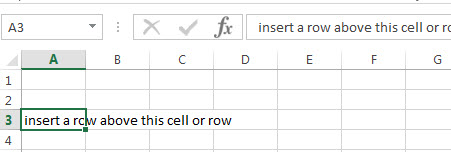Step 2# Right-click and then click “Insert…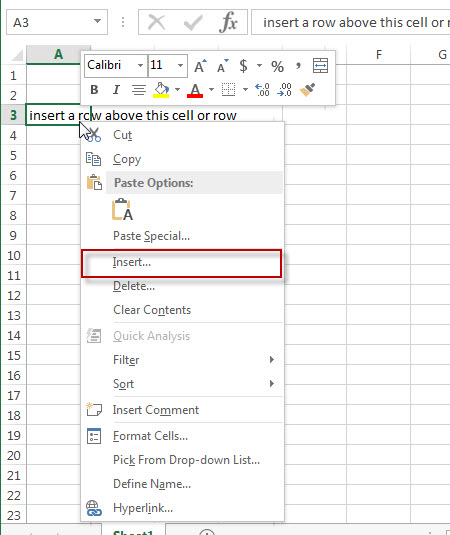Step 3# A “Insert” window will appear. Then click “Entire row” radio button.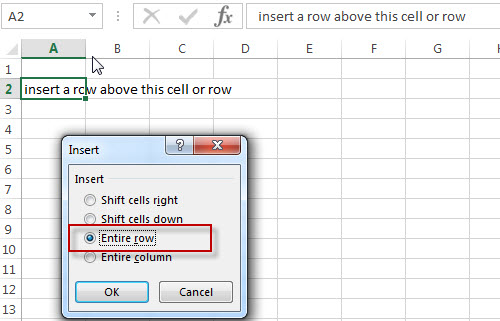Step 4# Let’ see the result.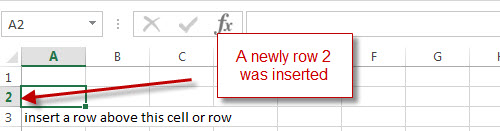## Delete Row

If you wish to delete one row or multiple rows in current workbook, just following below steps:

Step 1# Select a cell or a row that you want to delete. For example: select row 3 or A3 cell.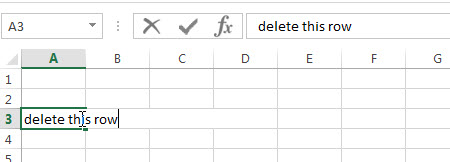Step 2# Right-click and then click “Delete…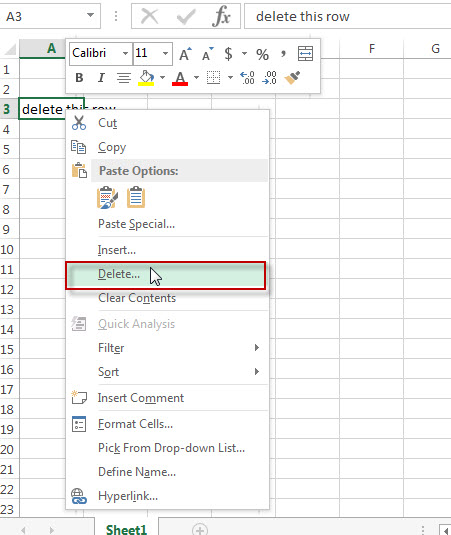Step 3# A “Delete” window will appear. Then click “Entire row” radio button. Click “OK” button.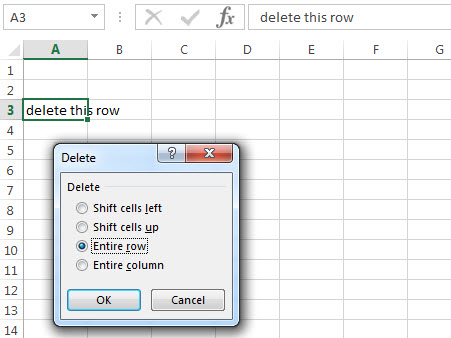Step 4# Let’ see the result.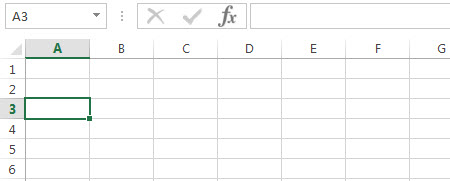Note: if you want to delete multiple rows, you just need to select those rows, then right-click on it.  next, click “Delete”. All rows you selected will be deleted immediately.

## Hide row

The below steps will explain that how to hide a row in excel.

Step 1# Select row that you wish to hide via clicking row number.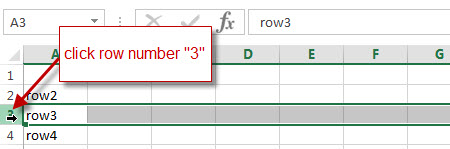Step 2# Right-click on row 3, then click “Hide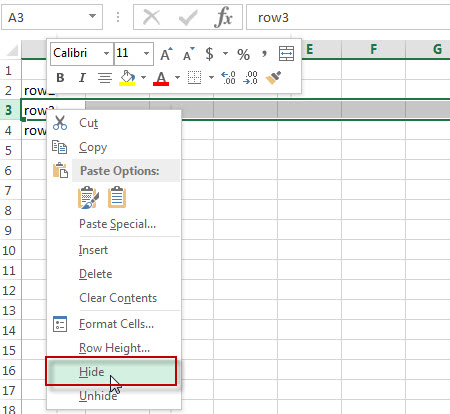Step 3# Let’s see the result, row 3 should be hidden.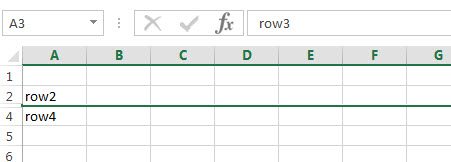## Unhide row

If you wish to unhide the hidden row, please refer to the below steps:

Step 1# Select the rows that contain the hidden row. For example, row 3 was hidden, now we need to select row 2 and row 4.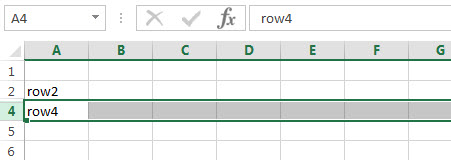Step 2# Right click and select “Unhide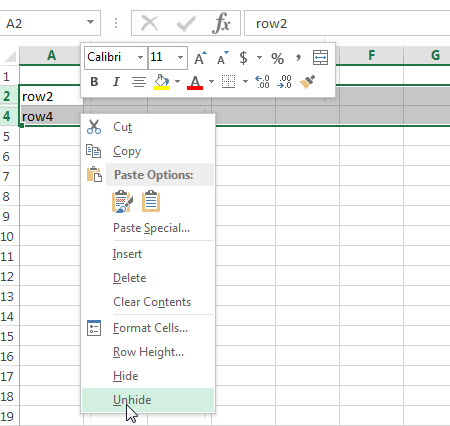Step 3# The hidden row should be unhidden, let’s see the result.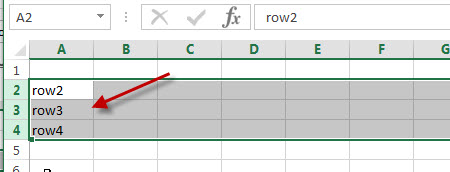## Sizing row

Sometimes, we wish to change the height of a row in excel, the below steps will guide you how to change the row height.

Step 1# Select row that you want to change height via clicking row number.

Step 2# Right-click and then click “Row Height…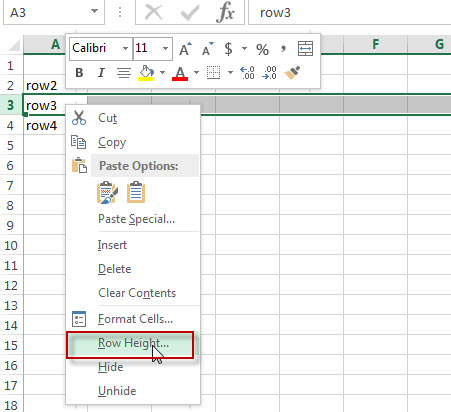Step 3# A “Row Height” window will appear.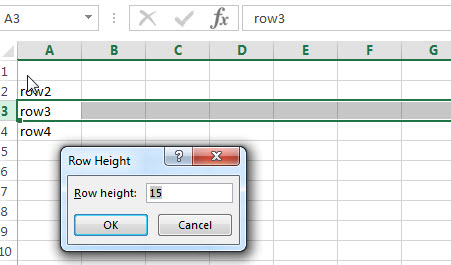Step 4# Input new value for Row height, such as: 30, then click “OK” button.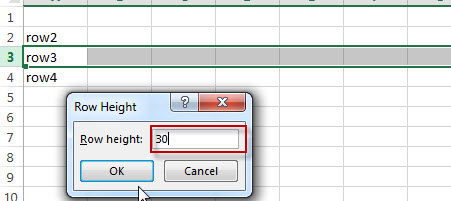Step 5# Let’s see the result. Row 3 should be the new row height.